Number 118 divide into two addends, so first addend is 69 greater than 75% of the second addend.

Correct result:

a =  90
b =  28

#### Solution:

a+b=118
a = 69+0.75 b

a+b=118
a = 69+0.75•b

a+b = 118
a-0.75b = 69

a = 90
b = 28

Our linear equations calculator calculates it.We would be pleased if you find an error in the word problem, spelling mistakes, or inaccuracies and send it to us. Thank you!Tips to related online calculators
Do you have a linear equation or system of equations and looking for its solution? Or do you have quadratic equation?

## Next similar math problems: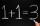Number 839 divide into the two addends that the first was 17 greater than 60% of the second. Determine these addends.
• Sum of three numbersThe sum of three numbers from which second number is 20% smaller than the first number and the third number is 25% smaller than the second number is 96. Determine this numbers.
• Apartments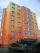Apartment on the first floor was 10% more expensive than the same apartment on the second floor. The difference was 105 Kc annually. Calculate the annual rent of the apartment in the first floor and from apartment on the second floor.
• Three piles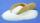100 kg of sugar we divide into three piles. The first pile is small. If we added to second 2 kg of sugar it would have 25% more sugar than the first pile. If we added to third pile 3 kg of sugar it would have 20% more sugar than the 2nd pile. How many kil
• Unknown number 10Number first increased by 30%, then by 1/5. What percentage we've increased the original number?
• 3 days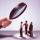Worker checked 2,950 products in 3 days. Second day checked 25% more than the first day. The third day 15% more products than the second day. How many products he checked in each day.
• Three workersThree workers were rewarded CZK 9200 and the money divided by the work they have done. First worker to get twice than the second, the second three times more than the third. How much money each worker received?
• Four numbersThe first number is 50% second, the second number is 40% third, the third number is 20% of the fourth. The sum is 396. What are the numbers?
• Container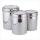First day got off 1/4 water from full container, second day 1/5 of the remaining water. How many percent remain container filled?
• Two workers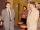Two workers make 138 parts/items. The first one produces 30% more than the second. How many items will each produce?
• Magic numberThe number 135 split to two addends so that one addend was 30 greater than 2/5 the addend.
• Three numbersFind three numbers so that the second number is 4 times greater than the first and the third is lower by 5 than the second number. Their sum is 67.
• Difference of two numberThe difference of two numbers is 20. They are positive integers greater than zero. The first number raised to one-half equals the second number. Determine the two numbers.
• Sales of productsFor 80 pieces of two quality products a total sales is 175 Eur. If the first quality product was sold for n EUR per piece (n natural number) and the second quality product after 2 EUR per piece. How many pieces of the first quality were sold?
• Crowns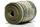1180 crowns is divided into three people that A got 20% less than B and C by 15% more than B. How many got A,B and C?
• Unknown number 5I think unknown number. If we enlarge it five times then subtract 3 and result decreases by 75% we get the number by one greater than the number I think. What number am I thinking?
• Three friends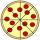Divide 570 euros to three friends so that first will get 50 euros less than the second and third twice more than the first. How many euros will get everyone?